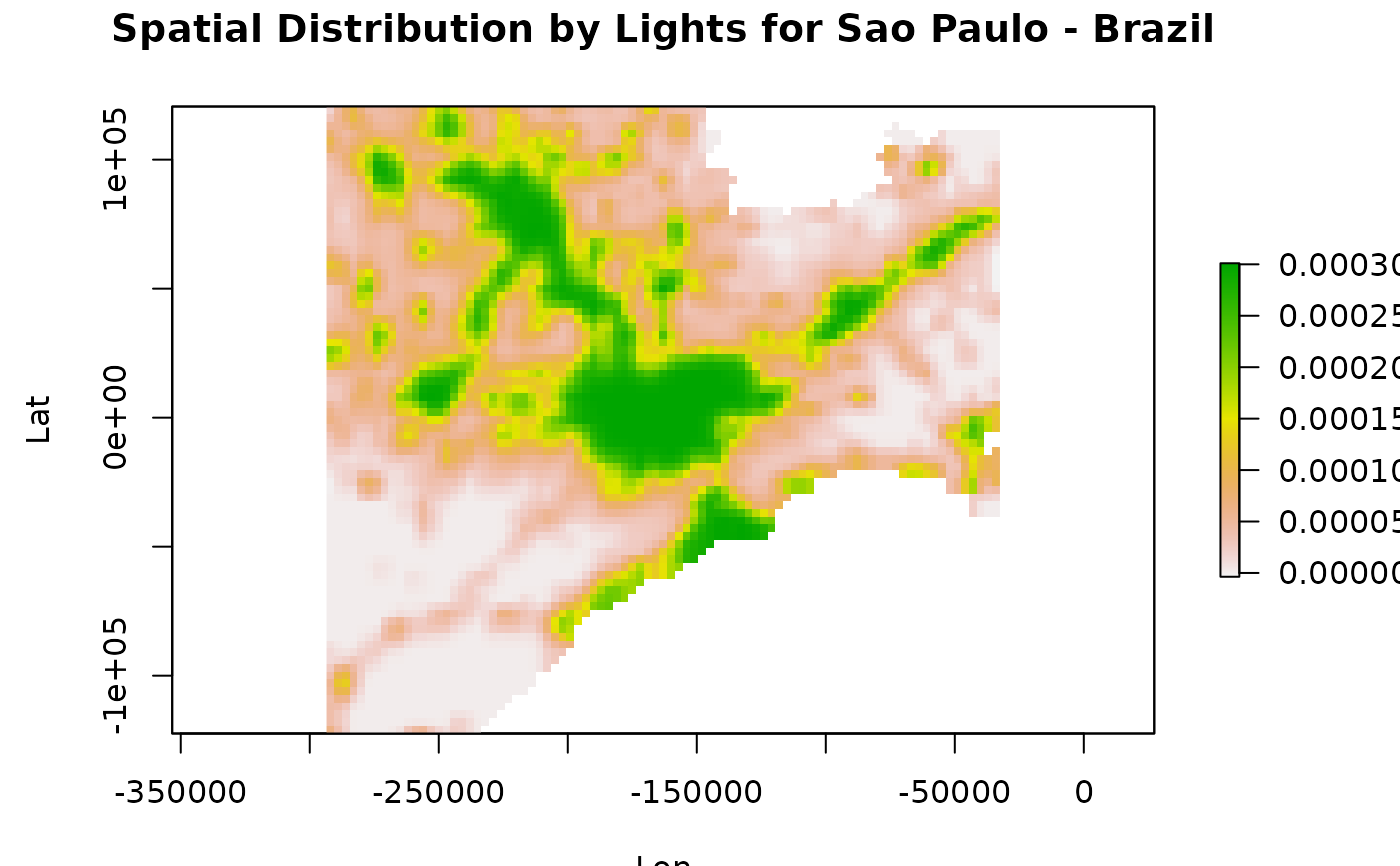Calculate the spatial distribution by a raster masked by shape/model grid information.

areaSource(s, r, grid = NA, name = "", as_frac = FALSE, verbose = TRUE)

## Source

Data avaliable https://www.ospo.noaa.gov/Operations/DMSP/index.html

## Arguments

s

input shape object

r

input raster object

grid

grid with the output format

name

area name

as_frac

return a fraction instead of the raster value

verbose

## Value

a raster object containing the spatial distribution of emissions

## Details

About the DMSP and example data https://en.wikipedia.org/wiki/Defense_Meteorological_Satellite_Program

## Examples

shape  <- raster::shapefile(paste(system.file("extdata", package = "EmissV"),
"/BR.shp",sep=""),verbose = FALSE)
shape  <- shape[22,1] # subset for Sao Paulo - BR
raster <- raster::raster(paste(system.file("extdata", package = "EmissV"),
"/dmsp.tiff",sep=""))
grid   <- gridInfo(paste(system.file("extdata", package = "EmissV"),"/wrfinput_d02",sep=""))
#> Grid information from: C:/Users/schuch/AppData/Local/Temp/Rtmp2H8oV2/temp_libpath3ae4412c5669/EmissV/extdata/wrfinput_d02
SP     <- areaSource(shape,raster,grid,name = "SPMA")
#> processing SPMA area ...
#> fraction of SPMA area inside the domain = 0.473725382325179
# \donttest{
sp::spplot(SP,scales = list(draw=TRUE),ylab="Lat",xlab="Lon",
main=list(label="Spatial Distribution by Lights for Sao Paulo - Brazil"),
col.regions = c("#031638","#001E48","#002756","#003062",
"#003A6E","#004579","#005084","#005C8E",
"#006897","#0074A1","#0081AA","#008FB3",
"#009EBD","#00AFC8","#00C2D6","#00E3F0"))# }